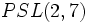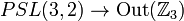# Groups of order 504

## Contents

See pages on algebraic structures of order 504| See pages on groups of a particular order

## Statistics at a glance

### Factorization and useful forms

The order 504 has prime factors 2, 3, and 7, with factorization:$504 = 2^3 \cdot 3^2 \cdot 7 = 8 \cdot 9 \cdot 7$

There are a number of useful forms for this number:$504 = 8^3 - 8 = 84(7 - 1)$

Quantity Value Explanation
Total number of groups up to isomorphism 202
Number of abelian groups up to isomorphism 6 (number of abelian groups of order$2^3$) times (number of abelian groups of order$3^2$) times (number of abelian groups of order$5^1$) =$3 \times 2 \times 1 = 6$. See classification of finite abelian groups and structure theorem for finitely generated abelian groups.
Number of nilpotent groups up to isomorphism 10 (number of groups of order 8) times (number of groups of order 9) times (number of groups of order 7) =$5 \times 2 \times 1 = 10$. See number of nilpotent groups equals product of number of groups of order each maximal prime power divisor, which in turn follows from equivalence of definitions of finite nilpotent group.
Number of supersolvable groups up to isomorphism 157
Number of solvable groups up to isomorphism 200 There are only two non-solvable groups: direct product of PSL(3,2) and Z3 (ID: (504,157))and projective special linear group:PSL(2,8) (ID: (504,156)). Of these, the latter is simple.
Number of simple non-abelian groups up to isomorphism 1 projective special linear group:PSL(2,8). It is also equal to$SL(2,8)$ and$PGL(2,8)$.

## Classification of non-solvable groups

First, we need to classify the possibilities for simple non-abelian composition factors. The only simple non-abelian groups of order dividing 504 are projective special linear group:PSL(3,2) (order 168, also isomorphic to$PSL(2,7)$) and projective special linear group:PSL(2,8) (order 504).

By order considerations, the possibilities for the composition factors are:

It remains to classify the groups with$PSL(3,2)$ and$\mathbb{Z}_3$ as its composition factors. It turns out that the only possibility is direct product of PSL(3,2) and Z3. We consider two (overlapping) possibilities:

The discussion below is related to supergroups of projective special linear group:PSL(3,2)
• There is a normal subgroup isomorphic to$PSL(3,2)$ and the quotient is cyclic of order three: In this, case, there is a mapping from the quotient group$\mathbb{Z}_3$ to the outer automorphism group of$PSL(3,2)$ via the conjugation action (see quotient group maps to outer automorphism group of normal subgroup). However, the outer automorphism group of$PSL(3,2)$ is isomorphic to cyclic group:Z2, which has no nontrivial homomorphisms from cyclic group:Z3. Thus, the outer action is trivial, and the whole group must therefore be an internal direct product, specifically, it must be direct product of PSL(3,2) and Z3.
• There is a normal subgroup isomorphic to$\mathbb{Z}_3$ and the quotient group is isomorphic to$PSL(3,2)$: Note first that the normal subgroup must be in the center because the induced map$PSL(3,2) \to \operatorname{Out}(\mathbb{Z}_3)$ must be trivial. The possibilities for this can be judged by looking at$H^2(PSL(3,2);\mathbb{Z}_3)$ for the trivial action. But, in fact,$PSL(3,2)$ has Schur multiplier$\mathbb{Z}_2$ and trivial abelianization, so by the dual universal coefficients for group cohomology, the cohomology group math>H^2(PSL(3,2);\mathbb{Z}_3)[/itex] is trivial. Thus, the only possible extension is direct product of PSL(3,2) and Z3.

## GAP implementation

The order 504 is part of GAP's SmallGroup library. Hence, any group of order 504 can be constructed using the SmallGroup function by specifying its group ID. Also, IdGroup is available, so the group ID of any group of this order can be queried.

Further, the collection of all groups of order 504 can be accessed as a list using GAP's AllSmallGroups function.

Here is GAP's summary information about how it stores groups of this order, accessed using GAP's SmallGroupsInformation function:

gap> SmallGroupsInformation(504);

There are 202 groups of order 504.
They are sorted by their Frattini factors.
1 has Frattini factor [ 42, 1 ].
2 has Frattini factor [ 42, 2 ].
3 has Frattini factor [ 42, 3 ].
4 has Frattini factor [ 42, 4 ].
5 has Frattini factor [ 42, 5 ].
6 has Frattini factor [ 42, 6 ].
7 - 11 have Frattini factor [ 84, 7 ].
12 - 18 have Frattini factor [ 84, 8 ].
19 - 21 have Frattini factor [ 84, 9 ].
22 has Frattini factor [ 84, 10 ].
23 has Frattini factor [ 84, 11 ].
24 - 28 have Frattini factor [ 84, 12 ].
29 - 33 have Frattini factor [ 84, 13 ].
34 - 38 have Frattini factor [ 84, 14 ].
39 - 41 have Frattini factor [ 84, 15 ].
42 has Frattini factor [ 126, 7 ].
43 has Frattini factor [ 126, 8 ].
44 has Frattini factor [ 126, 9 ].
45 has Frattini factor [ 126, 10 ].
46 has Frattini factor [ 126, 11 ].
47 has Frattini factor [ 126, 12 ].
48 has Frattini factor [ 126, 13 ].
49 has Frattini factor [ 126, 14 ].
50 has Frattini factor [ 126, 15 ].
51 has Frattini factor [ 126, 16 ].
52 has Frattini factor [ 168, 43 ].
53 has Frattini factor [ 168, 44 ].
54 has Frattini factor [ 168, 45 ].
55 has Frattini factor [ 168, 46 ].
56 has Frattini factor [ 168, 47 ].
57 has Frattini factor [ 168, 48 ].
58 has Frattini factor [ 168, 49 ].
59 has Frattini factor [ 168, 50 ].
60 has Frattini factor [ 168, 51 ].
61 has Frattini factor [ 168, 52 ].
62 has Frattini factor [ 168, 53 ].
63 has Frattini factor [ 168, 54 ].
64 has Frattini factor [ 168, 55 ].
65 has Frattini factor [ 168, 56 ].
66 has Frattini factor [ 168, 57 ].
67 - 73 have Frattini factor [ 252, 26 ].
74 has Frattini factor [ 252, 27 ].
75 - 79 have Frattini factor [ 252, 28 ].
80 - 84 have Frattini factor [ 252, 29 ].
85 - 89 have Frattini factor [ 252, 30 ].
90 has Frattini factor [ 252, 31 ].
91 has Frattini factor [ 252, 32 ].
92 - 98 have Frattini factor [ 252, 33 ].
99 - 105 have Frattini factor [ 252, 34 ].
106 - 110 have Frattini factor [ 252, 35 ].
111 - 117 have Frattini factor [ 252, 36 ].
118 - 122 have Frattini factor [ 252, 37 ].
123 - 125 have Frattini factor [ 252, 38 ].
126 has Frattini factor [ 252, 39 ].
127 has Frattini factor [ 252, 40 ].
128 - 132 have Frattini factor [ 252, 41 ].
133 - 137 have Frattini factor [ 252, 42 ].
138 - 142 have Frattini factor [ 252, 43 ].
143 - 147 have Frattini factor [ 252, 44 ].
148 - 152 have Frattini factor [ 252, 45 ].
153 - 155 have Frattini factor [ 252, 46 ].
156 - 202 have trivial Frattini subgroup.

For the selection functions the values of the following attributes
are precomputed and stored:
IsAbelian, IsNilpotentGroup, IsSupersolvableGroup, IsSolvableGroup,
LGLength, FrattinifactorSize and FrattinifactorId.

This size belongs to layer 2 of the SmallGroups library.
IdSmallGroup is available for this size.